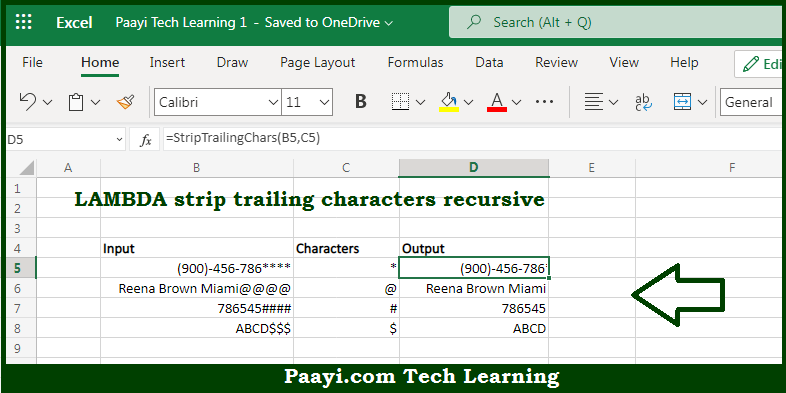# Learn How to LAMBDA Strip Trailing Characters Recursive in Microsoft Excel

Written by | 0 Comments | 414 Views

In this article, you will learn how to evaluate things in Dynamic Arrays with formulas in Microsoft Excel using a single/combination(s) of functions. You will also know How to LAMBDA Strip Trailing Characters Recursive and see the generic formula.

Learn How to LAMBDA Strip Trailing Characters Recursive in Microsoft Excel

The main purpose of this formula is to strip trailing characters recursive. Here we will learn how to strip trailing characters recursive in the given data range in the workbook in Microsoft Excel. That implies, with the help of a formula based on the LAMBDA function you can able to strip trailing characters recursive. So, with the help of this formula, you can able to strip trailing characters recursive in the given data range in the workbook in Microsoft Excel.

General Formula to LAMBDA Strip Trailing Characters Recursive

=LAMBDA(str,char,IF(RIGHT(str)<>char,str,StripTrailingChars(MID(str,1,LEN(str)-1),  char)))

The Explanation for LAMBDA Strip Trailing Characters RecursiveSo we know that with the help of the given formula above you can able to strip trailing characters recursive. Here we will learn how to strip trailing characters recursive in the given data range in the workbook in Microsoft Excel. As we know that the while creating a recursive LAMBDA formula the main goal is how the formula will "exit" the loop it performs by calling itself. Here, before the recursive call happens, the IF function is used to check the last character of the input text string. So, with the help of this formula, you can able to strip trailing characters recursive. Here we will learn how to strip trailing characters recursive in the given data range in the workbook in Microsoft Excel.